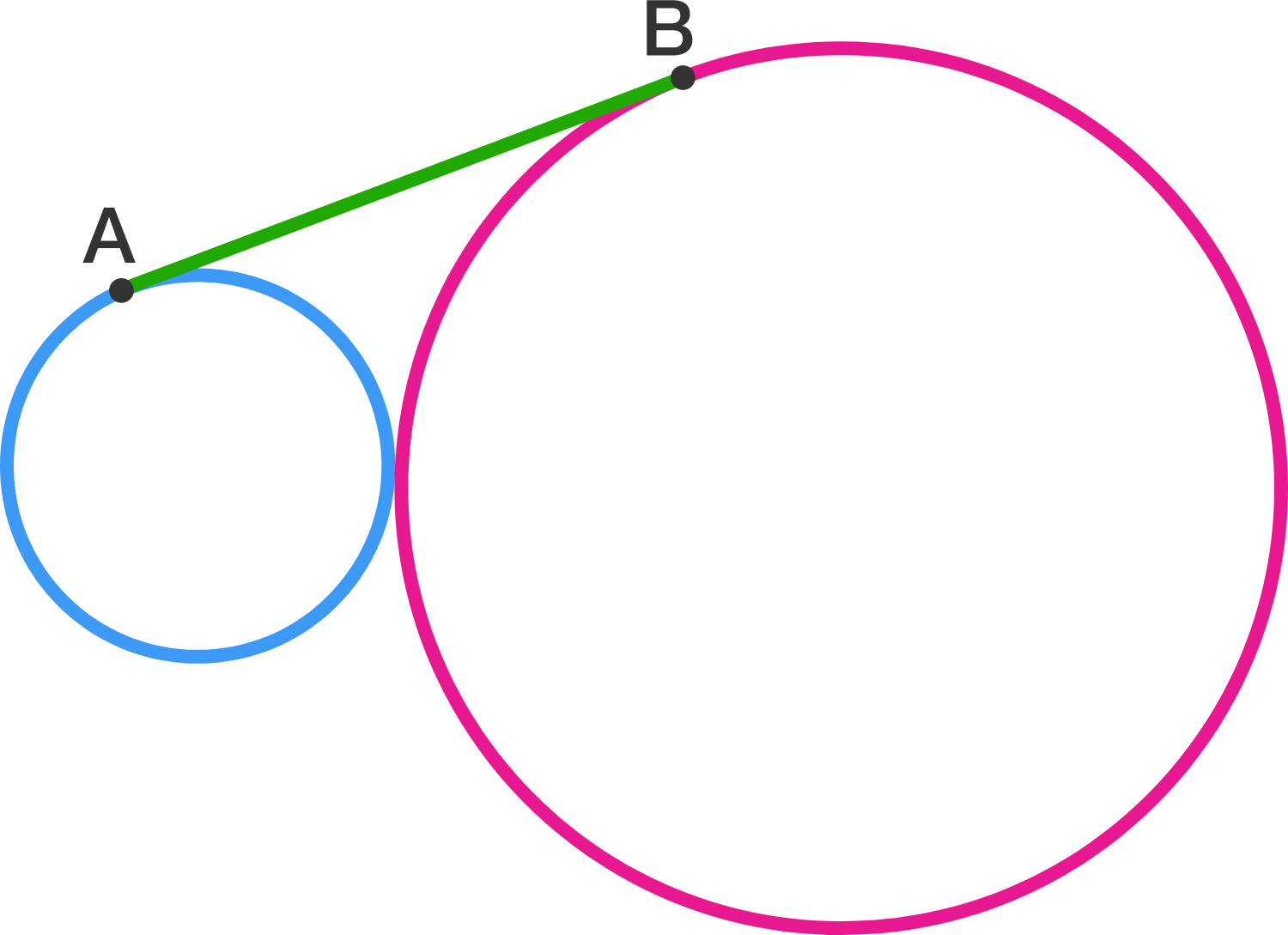# Tangent circles

Geometry Level 4Two circles are tangent to each other, and their diameters $d_1$ and $d_2$ satisfy $d_1 \times d_2=36$.
Segment $AB$ is tangent to both circles. Find the length of $AB$.

If you think that the length of $AB$ depends on the relative values of $d_1$ and $d_2$, submit your answer as 9999.

×